Home Practice
For learners and parents For teachers and schools
Textbooks
Full catalogue
Pricing SupportLog in

We think you are located in United States. Is this correct?

# Solving more geometric problems

## 12.6 Solving more geometric problems

When solving geometry problems involving lines, you need to use some facts about quadrilaterals and triangles.

• The sum of the interior angles of a triangle is $$180^\circ$$.
• The exterior angle of a triangle is equal to the sum of the interior opposite angles.
exterior angle of a triangle
the angle formed outside a triangle when one of its sides is produced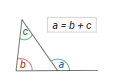• The interior angles of a quadrilateral add up to $$360^{\circ}$$.
$$\hat{P}+\hat{Q}+\hat{R}+\hat{S}= 360^{\circ}$$
interior angle
an angle that lies inside a shape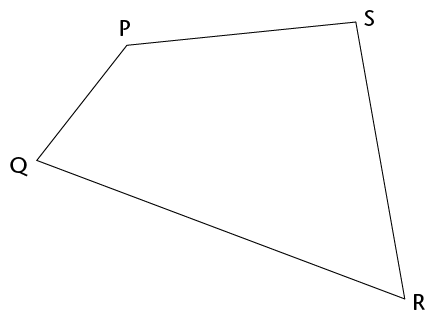## Worked example 12.9: Identifying angles on parallel lines

Calculate the size of angles $$a$$, $$b$$, $$c$$ and $$d$$.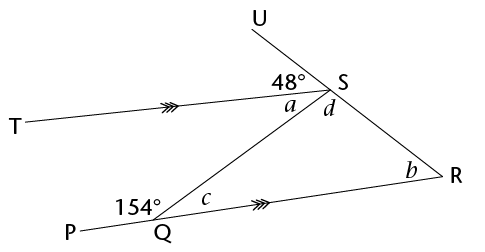### Examine the parallel lines. Identify how many transversals there are.

Notice that there are two traversals: $$SQ$$ and $$UR$$. Now look for the angles that form F or Z pattern along each transversal.

• Transversal $$UR$$: We can see that $$b$$ is a corresponding angle to $$48^{\circ}$$, making an F pattern. There are no alternate angles formed by this transversal.
• Transversal $$SQ$$: We can see that $$a$$ is alternate to $$c$$, making a Z pattern. There are no corresponding angles formed by this transversal.

Angle $$c$$ is an adjacent supplementary angle to 154°.

\begin{align} b & = 48^{\circ}&&(\text{corresp }\angle\text{s; } TS \parallel PR) \\ c & = 180^{\circ} - 154^{\circ}&&(\angle\text{s on a straight line}) \\ c & = 26^{\circ} \\ a & = 26^{\circ}&&(\text{alt }\angle\text{s; } TS \parallel PR) \\ d & = 180^{\circ}-48^{\circ} - 26^{\circ} &&(\angle\text{s on a straight line}) \\ d & = 106^{\circ} \end{align}

## Worked example 12.10: Using angles on parallel lines

Calculate the size of $$x$$.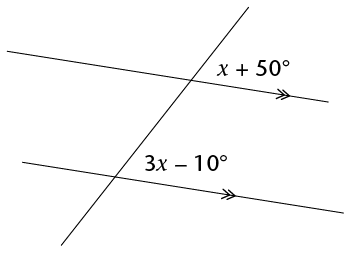### The two given angles lie between parallel lines, and are corresponding angles, therefore they are equal. We will use this fact to form an equation to help us solve for $$x$$.

\begin{align} x+50^{\circ} &= 3x-10^{\circ} &&(\text{corresp }\angle\text{s}) \\ x-3x &= -10^{\circ} -50^{\circ} \\ -2x &= -60^{\circ} \\ x &= 30^{\circ} \end{align}

## Worked example 12.11: Using angles on parallel lines

Calculate the size of $$x$$.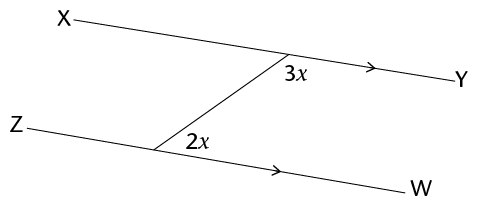### Notice that there is only one transversal in this diagram. The two given angles make a C pattern.

The two angles form co-interior angles, which add up to $$180^{\circ}$$, because the lines are parallel. So we can write out an equation using this information.

\begin{align} 2x+3x &= 180^{\circ} &&(\angle\text{s on a straight line}) \\ 5x &= 180^{\circ} \\ x &= 30^{\circ} \\ \end{align}

## Worked example 12.12: Solving geometric problems involving properties of triangles

Calculate the sizes of $$\hat{1}$$ to $$\hat{6}$$.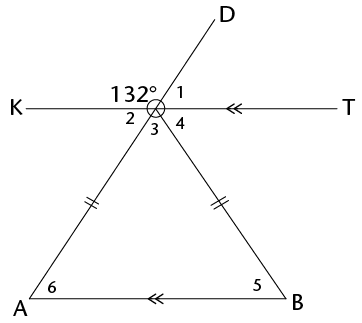### Examine the diagram carefully. Look for the parallel lines and all transversals.

Notice that there are two transversals; $$AD$$ and $$OB$$:

• Looking at $$AD$$, notice that $$\hat{2}$$ and $$\hat{6}$$ are alternate angles and therefore equal. We also see that $$\hat{1}$$ and $$\hat{6}$$ are corresponding angles and therefore equal.
• Looking at $$OB$$, we notice that $$\hat{4}$$ and $$\hat{5}$$ are alternate angles.

### Look for all the adjacent angles on a straight line.

You may need to use the fact they are supplementary later in your calculation. How many supplementary angles can you find?

The supplementary angles are:

\begin{align} 132^\circ+\hat{2}&=180^\circ \\ 132° + \hat{1}&=180^\circ \\ \hat{2}+\hat{3} + \hat{4}&=180^\circ \\ \hat{1} + \hat{3}+ \hat{4}&=180^\circ \end{align}

### Look for vertically opposite angles.

How many can you find? ($$\hat{1}$$ is vertically opposite $$\hat{2}$$).

\begin{align} \hat{1}&= 180^{\circ} -132^{\circ} &&(\angle\text{s on a straight line}) \\ \hat{1}&= 48^{\circ} \\ \hat{2}&= 48^{\circ} &&(\text{vert opp }\angle\text{s}) \\ \hat{6}&= 48^{\circ} &&(\text{alt }\angle\text{s; KT} \parallel {AB}) \end{align}

Hint: Remember that in an isoceles triangle, two sides are the same length.

Because $$\triangle ABO$$ is an isoceles triangle:

\begin{align} \hat{5}&= 48^{\circ} &&(\angle\text{s equal opp sides}) \\ \hat{3} &= 180^{\circ}- \hat{5}- \hat{6} &&(\angle\text{s in a }\triangle) \\ \hat{3} &= 180^{\circ}-48^{\circ}-48^{\circ} \\ \hat{3} &= 84^{\circ} \end{align}

## Worked example 12.13: Solving geometric problems involving properties of triangles and quadrilaterals

$$ABCD)\ is a parallelogram. Calculate the sizes of \(A\hat{B}D$$, $$A\hat{D}B$$, $$\hat{C}$$ and $$C\hat{B}D$$.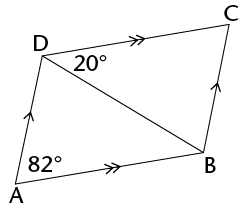### Use the properties of the parallelogram $$ABCD$$ to expand the given information.

Both pairs of opposite angles are equal in a parallelogram, therefore we can say that $$\hat{A}=\hat{C}$$.

### Look for any alternate or corresponding angles for both sets of parallel lines. We also want to make of a note of any co-interior angles formed by the parallel lines. These supplementary angles may also be useful in helping us to find the missing angles.

We are given two sets of parallel lines: $$AD \parallel BC$$ and $$DC \parallel AB$$.

• $$DC \parallel AB$$: Looking at transversal $$BD$$, we notice that $$20^{\circ}$$ and $$\hat{DBA}$$ are alternate angles formed by these parallel lines, and therefore are equal.
• $$AD \parallel BC$$: Looking at transversal $$BD$$, we notice that $$\hat{ADB}$$ and $$\hat{DBC}$$ are alternate angles formed by these parallel lines, and therefore are equal.

### Use the properties of triangles to find angles.

We are also given two triangles: $$\triangle{ABD}$$ and $$\triangle{DBC}$$. We can use the fact that the sum of $$\angle$$s of a $$\triangle$$ = $$180^\circ$$ to help find remaining angles.

\begin{align} \hat{ABD}&= 20^{\circ} &&(\text{alt }\angle\text{s; AB} \parallel {DC}) \\ \hat{ADB}&= 180^{\circ} -20^{\circ}-82^{\circ} &&(\angle\text{s in a }\triangle) \\ \hat{ADB}&= 78^{\circ} \\ \hat{C} &= 82^{\circ} &&(\text{opp sides of parallelogram}) \\ \hat{CBD}&= 78^{\circ} &&(\text{alt }\angle\text{s; AB} \parallel {DC}) \end{align}
Exercise 12.7

$$RSTU$$ is a trapezium. Calculate the sizes of $$\hat{T}$$ and $$\hat{R}$$.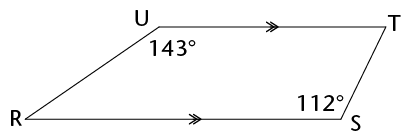\begin{align} \hat{R} &=180°-143° &&(\text{co-int}\angle\text{s}; UT \parallel RS) \\ \hat{R} &= 37° \\ \hat{T} &=180°-112° &&(\text{co-int}\angle\text{s}; UT \parallel RS) \\ \hat{T} &= 68° \end{align}

$$JKLM$$ is a rhombus. Calculate the sizes of of $$J\hat{M}L$$, $$\hat{M}_2$$ and $$\hat{K}_1$$.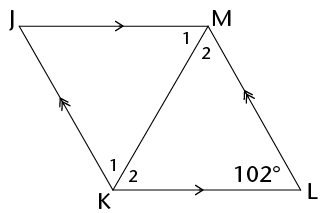Hint: Remember, $$JKLM$$ is a rhombus, therefore all four sides of $$JKLM$$ are equal.

\begin{align} \hat{J} &=102° &&(\text{opp }\angle\text{s of rhombus)} \\ \hat{M_2} &= \hat{K_2} &&(\text{opp }\angle\text{s; } \text { opp = sides; ML = KL}) \\ \hat{M_2} + \hat{K_2} +102° &= 180° \\ \hat{M_2} + \hat{K_2} +102° &= 180° - 102° \\ \hat{M_2} + \hat{K_2} +102° &= 78° \\ \hat{M_2} = \hat{K_2} &= 39° \\ \text{Similarly, } \hat{M_1} = \hat{K_1} &= 39° \\ J\hat{M}L &= \hat{M_1} + \hat{M_2} \\ &= 78° \end{align}

In the diagram, $$AB \parallel CD$$. Calculate the sizes of $$F\hat{H}G, \hat{F},\hat{C}$$ and $$\hat{D}$$. Give reasons for your answers.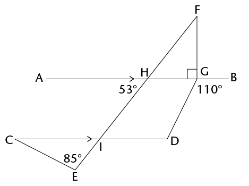\begin{align} \hat{FHG} &=53° && (\text{vert opp }\angle\text{s}) \\ \hat{F}+ \hat{FHG}+ 90° &=180°&&(\text{sum of }\angle\text{s in } \triangle )\\ \hat{F}+53°+ 90° &=180° \\ \hat{F}&=180° -90°-53° \\ \hat{F}&=37° \\ \\ \hat{CIE} &= 53° &&(\text{corresp }\angle\text{s}; AB \parallel CD) \\ \hat{C}+ \hat{E}+ \hat{CIE} &=180°&&(\text{sum of }\angle\text{s in } \triangle)\\ \hat{C}+85°+53° &=180° \\ \hat{C}&=180° -85°-53° \\ \hat{C}&=42° \\ \\ \hat{AGD} &= 180°-110 &&(\angle\text{s on a str line})\\ \hat{AGD} &= 70° \\ \hat{D} &= 180°-70° &&(\text{co-int }\angle\text{s}; AB \parallel CD) \\ \hat{D} &= 110° \end{align}